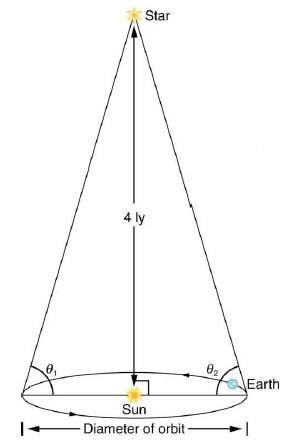Chapter 34, Problem 21PE

Chapter
Section
Textbook Problem

Construct Your Own ProblemConsider a star moving in a circular orbit at the edge at a galaxy. Construct a problem in which you calculate the mass of that galaxy in kg and in multiples of the solar mass based on the velocity of the star and its distance from the center of the galaxy.Figure 34.26 Distances to nearby stars are measured using triangulation, also called the parallax method. The angle of line of sight to the star is measured at intervals six momma apart, and the distance is calculated by using the known diameter of the Earth's orbit. This can be done for stars up to about 500 ly away.

To determine

The construction of the question by considering a star moving in a circular orbit at the edge of the galaxy and calculate the mass of the galaxy.

Explanation

Given:

Mass of Sun, ms=1.989×1030kg

Speed of light, v=3×108m/s

Gravitational constant, G=6.67×1011N-m2/kg2

Formula used:

Formula to calculate the mass of the galaxy,

M=v2rG ...... (I)

Where,

• v is the speed of the sun around the galaxy.
• M is mass of the star.
• G is gravitational constant.
• r is the radius of the sun.

Calculation:

Problem:

Calculate the mass of the galaxy and in multiple of the solar mass when a star having a radius equal to the radius of the sun moves around the center of the galaxy.

Since, the star is moving around the center of the galaxy with the speed equal to the speed of the light.

Substitute 3×108m/s for v , 6.67×1011N-m2/kg2 for G , and 30000ly for r in equation (I)

Still sussing out bartleby?

Check out a sample textbook solution.

See a sample solution

The Solution to Your Study Problems

Bartleby provides explanations to thousands of textbook problems written by our experts, many with advanced degrees!

Get Started

What are the moral implications of using ergogenic aids?

Understanding Nutrition (MindTap Course List)

What are sister chromatids?

Human Heredity: Principles and Issues (MindTap Course List)

Explain why ATP is referred to as the primary energy carrier?

Chemistry for Today: General, Organic, and Biochemistry